# Selina Solutions Concise Maths Class 10 Chapter 11 Geometric Progression

A sequence, in which each of its terms can be obtained by multiplying or dividing its preceding term by a fixed quantity, is called a geometric progression. So, this chapter is going to be completely about G.P., its general term, properties and the sum of terms in a G.P. Students who find it difficult to solve problems in this chapter can refer Selina Solutions for Class 10 Mathematics prepared by our experienced faculty at BYJU’S. The solutions are prepared with an aim to boost confidence among students for taking up their Class 10 final exams. This mainly improves problem-solving skills of the students, which are vital from an examination point of view. The Selina Solutions for Class 10 Mathematics Chapter 11 Geometric Progression PDF are available right below.

## Selina Solutions Concise Maths Class 10 Chapter 11 Geometric Progression Download PDF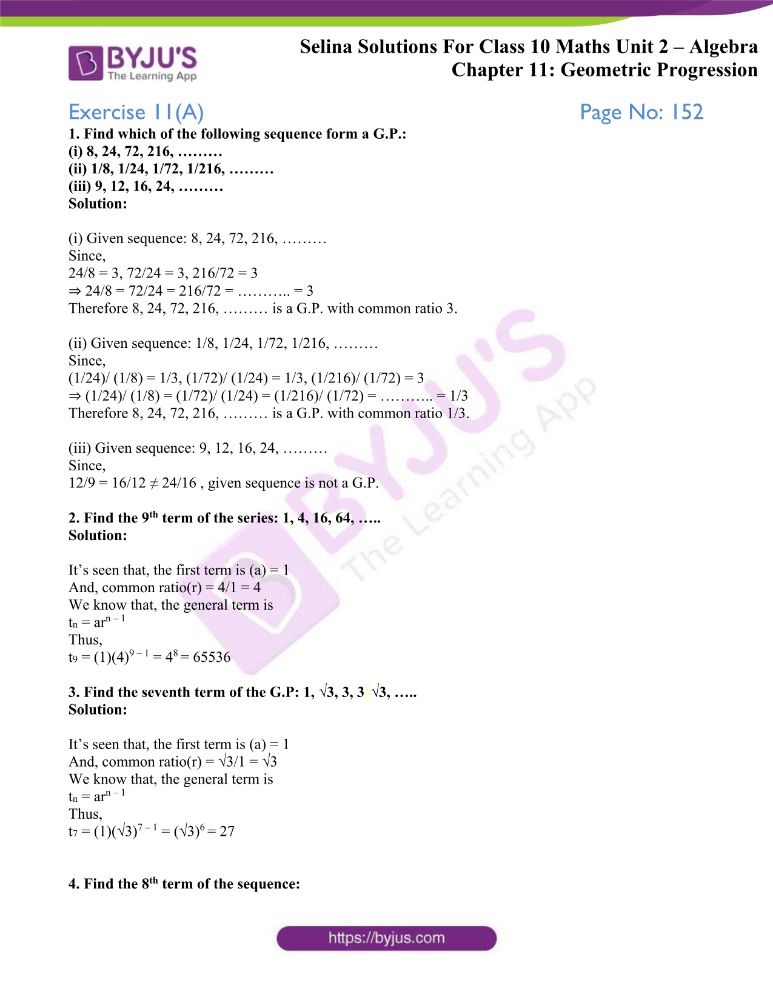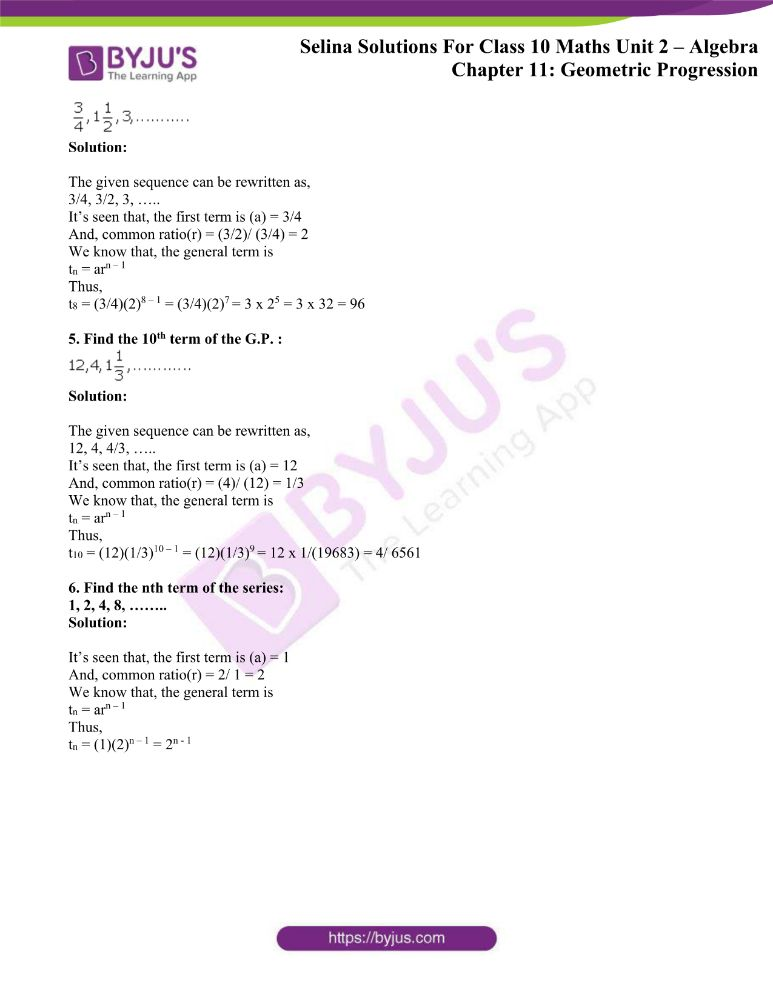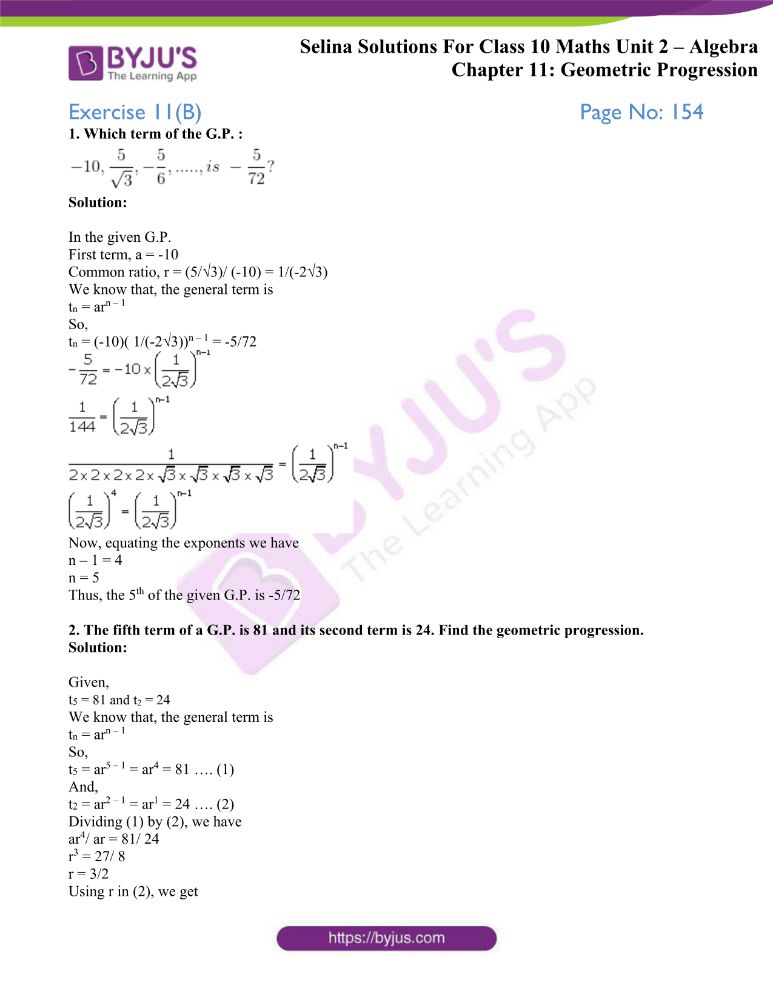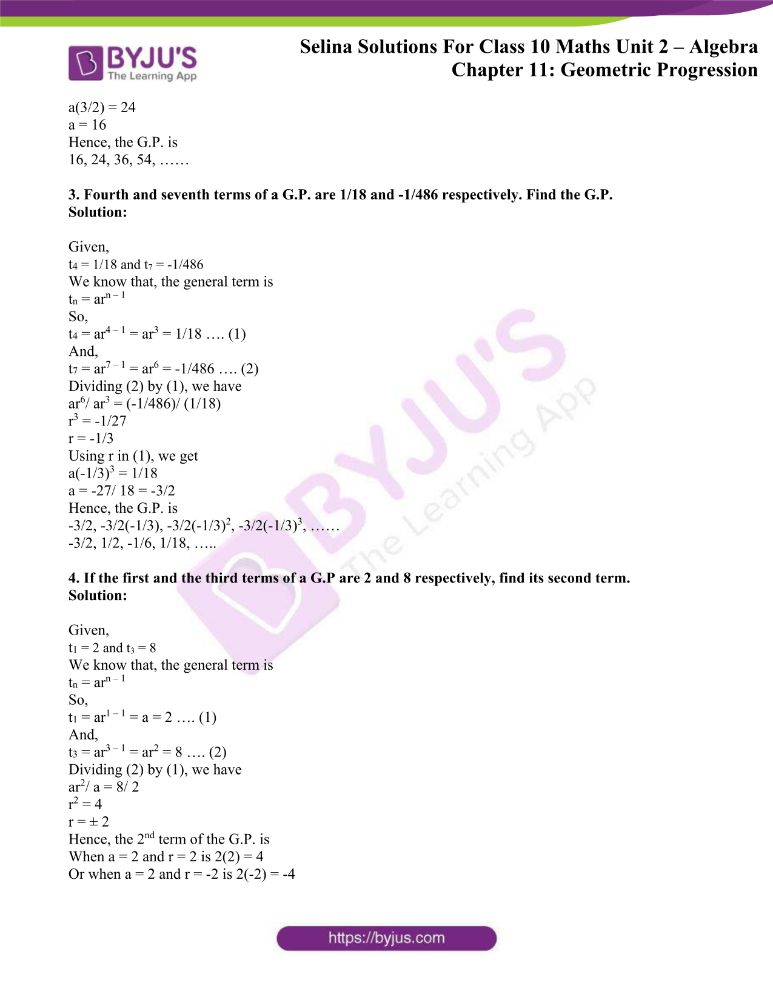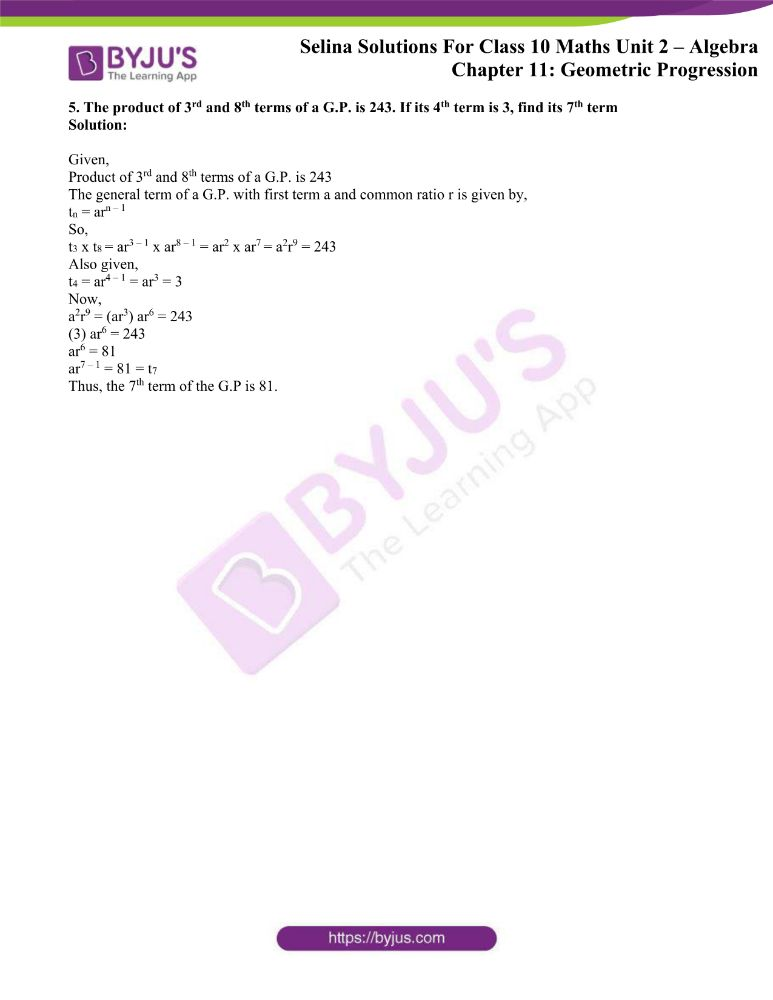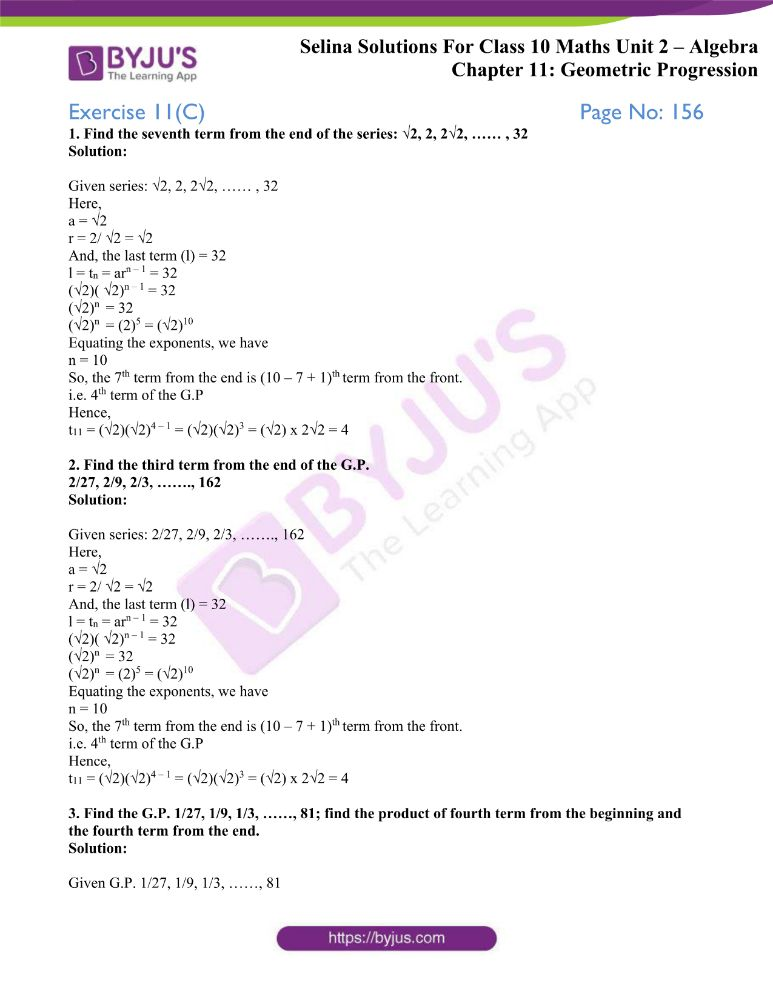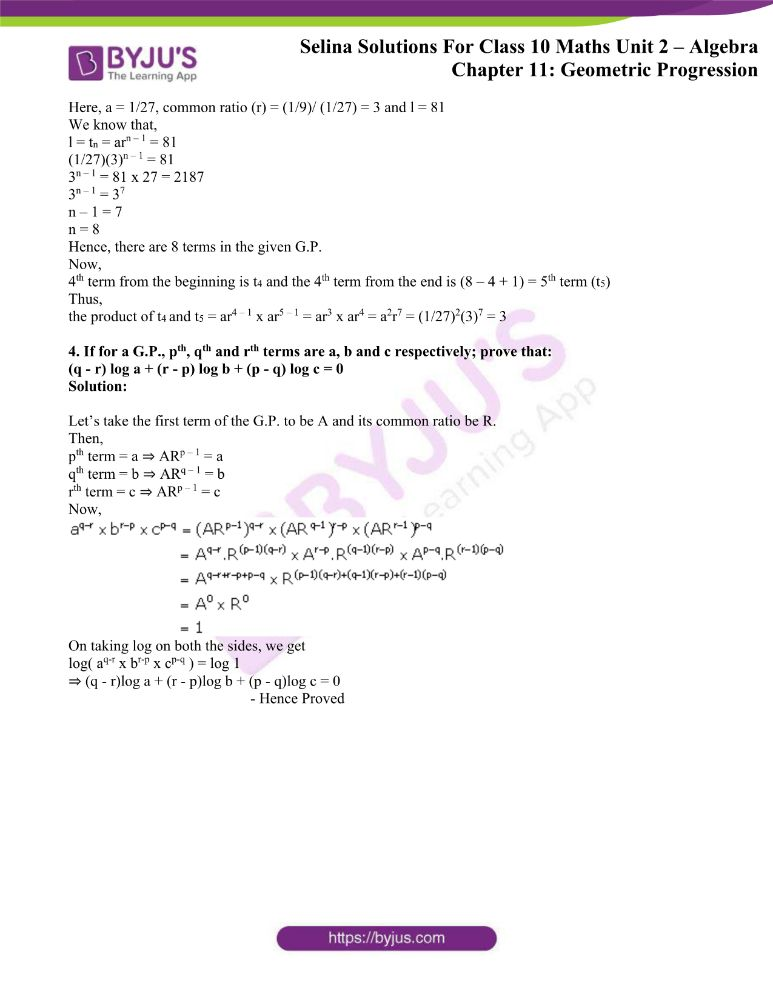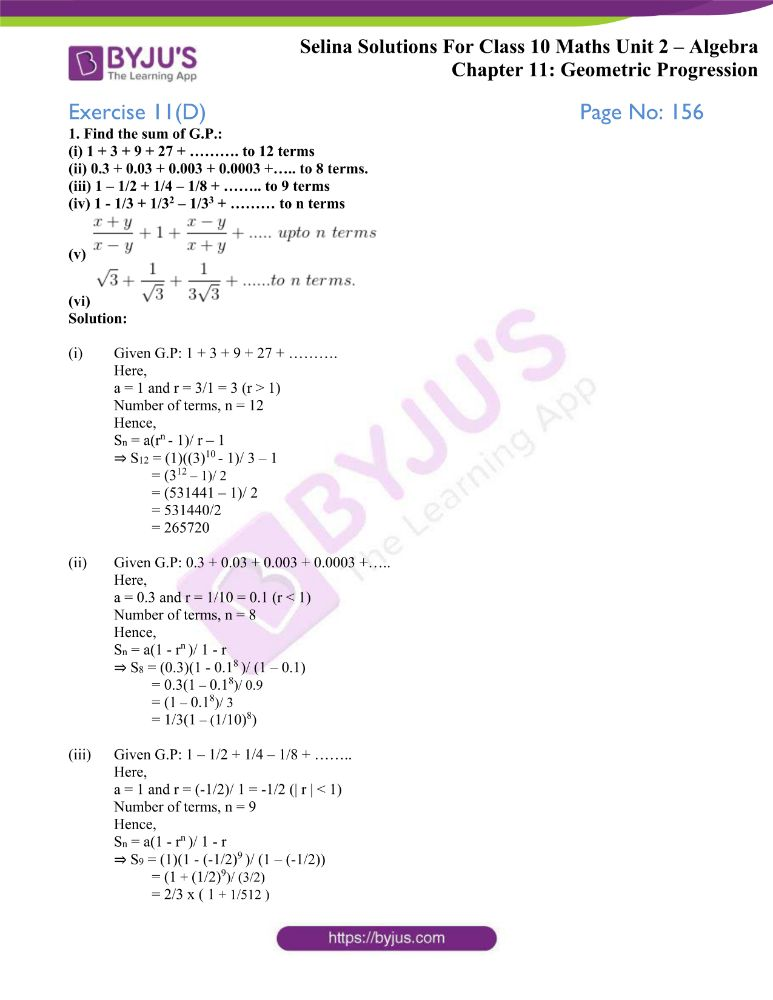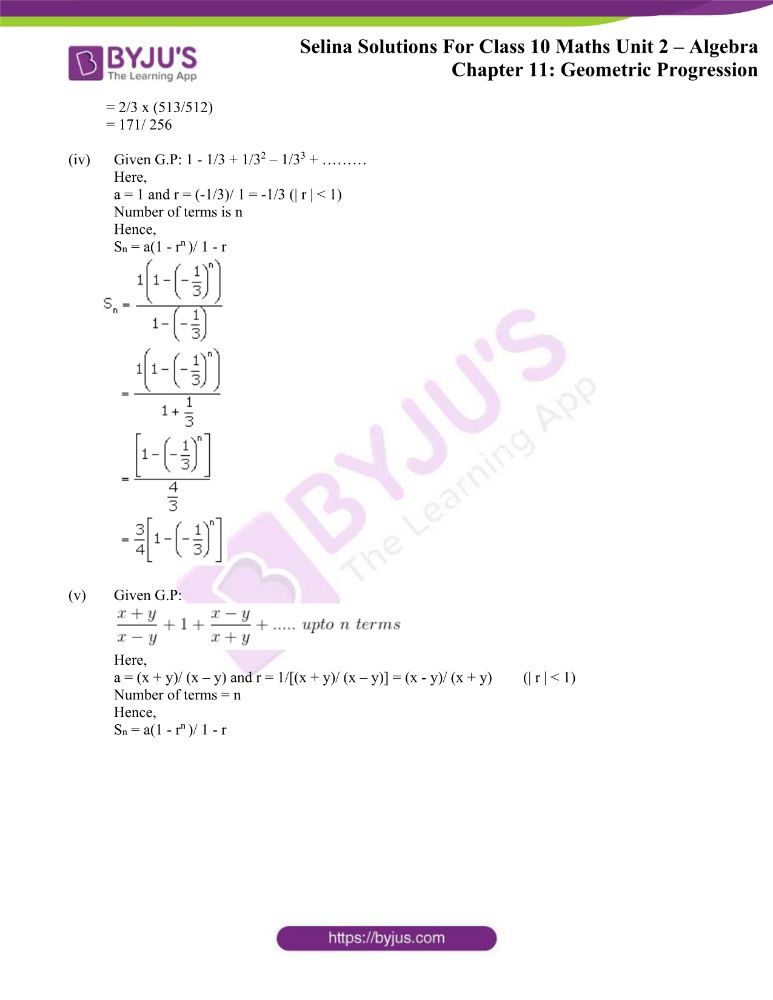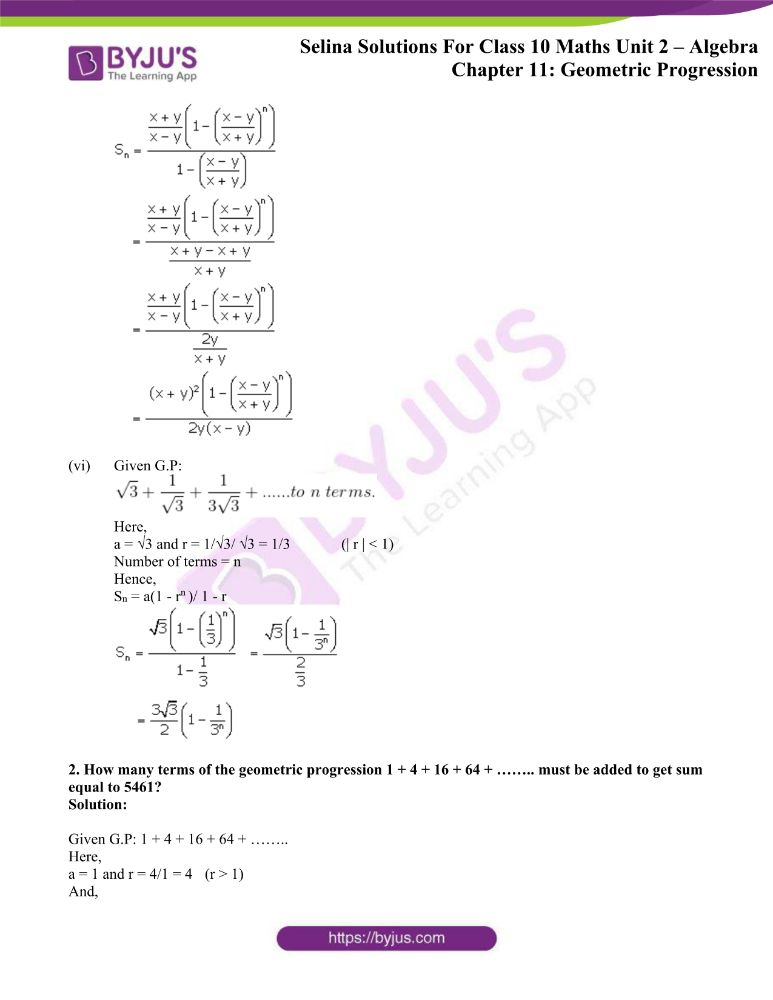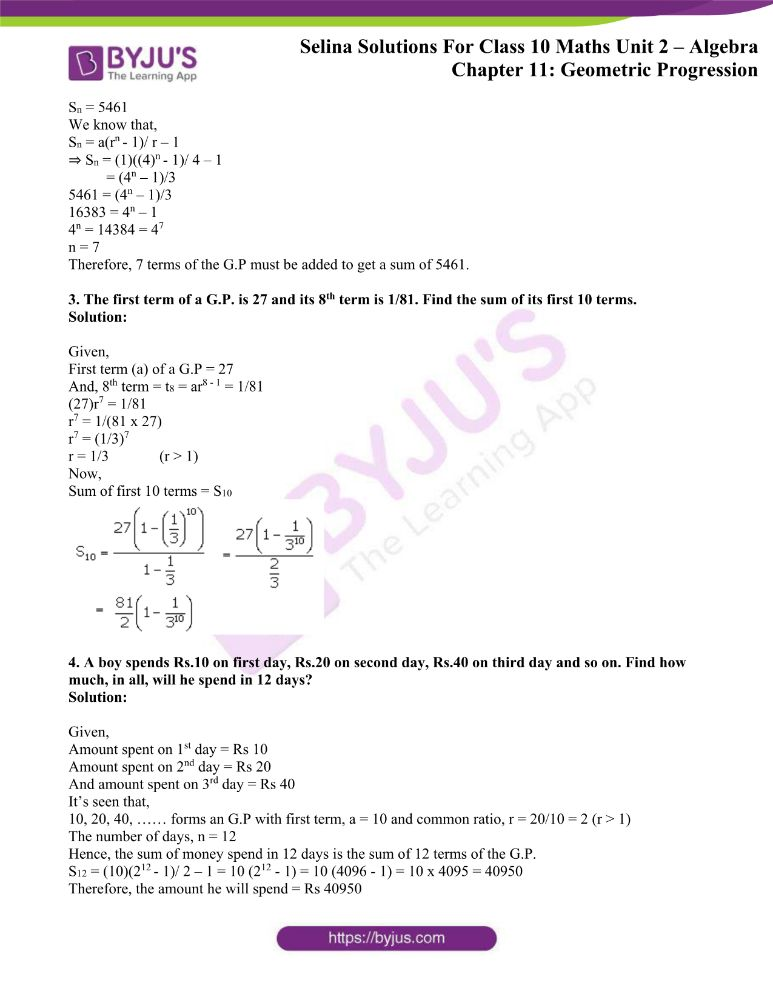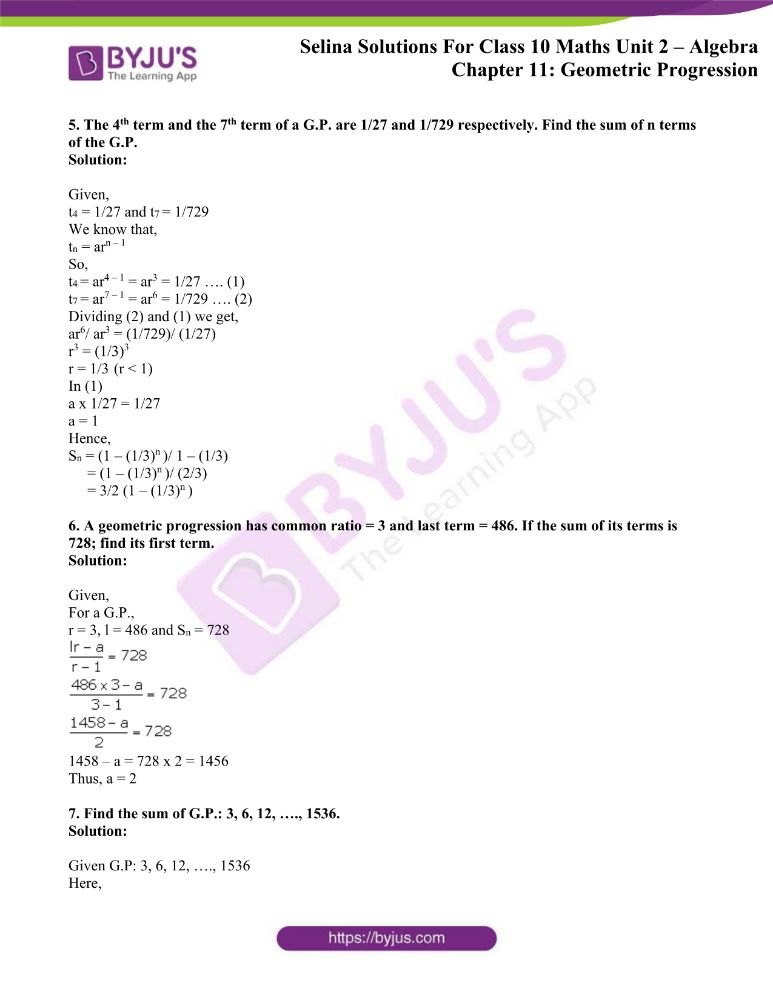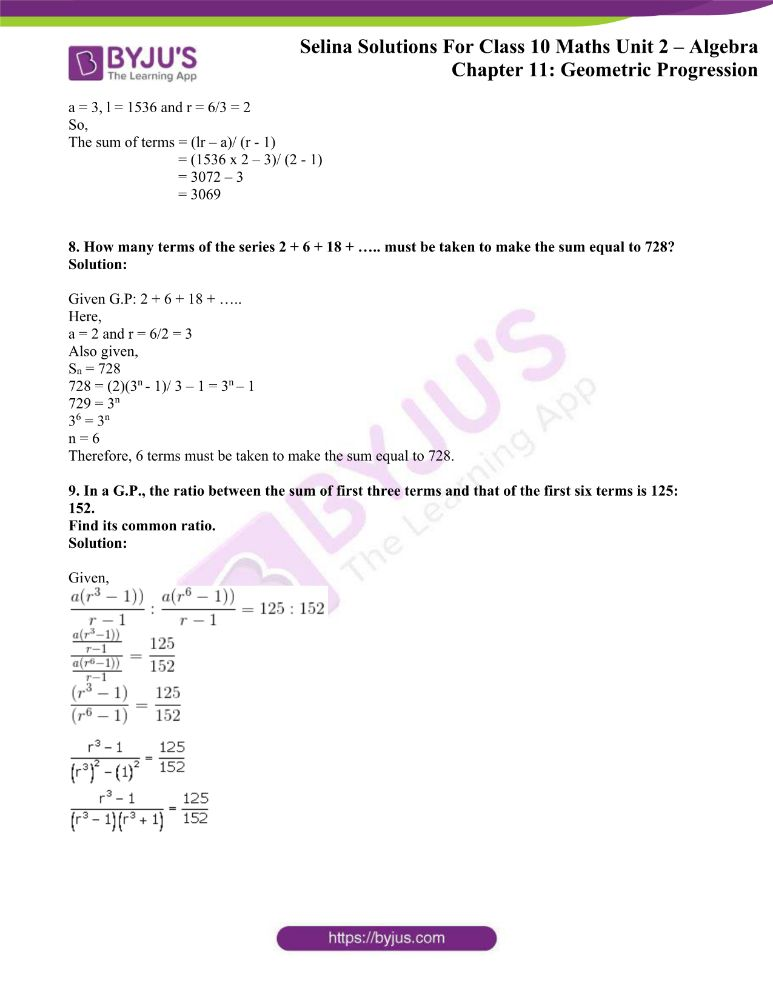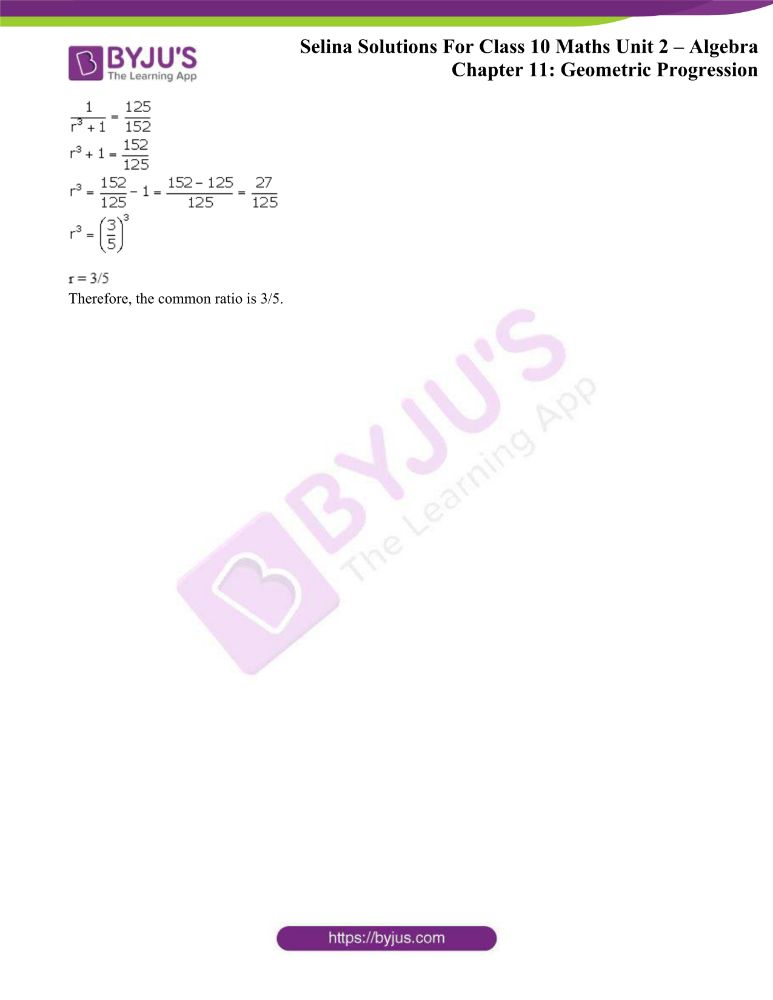### Exercises of Concise Selina Solutions Class 10 Maths Chapter 11 Geometric Progression

Exercise 11(A) Solutions

Exercise 11(B) Solutions

Exercise 11(C) Solutions

Exercise 11(D) Solutions

## Access Selina Solutions Concise Maths Class 10 Chapter 11 Geometric Progression

Exercise 11(A) Page No: 152

1. Find which of the following sequence form a G.P.:

(i) 8, 24, 72, 216, ………

(ii) 1/8, 1/24, 1/72, 1/216, ………

(iii) 9, 12, 16, 24, ………

Solution:

(i) Given sequence: 8, 24, 72, 216, ………

Since,

24/8 = 3, 72/24 = 3, 216/72 = 3

⇒ 24/8 = 72/24 = 216/72 = ……….. = 3

Therefore 8, 24, 72, 216, ……… is a G.P. with common ratio 3.

(ii) Given sequence: 1/8, 1/24, 1/72, 1/216, ………

Since,

(1/24)/ (1/8) = 1/3, (1/72)/ (1/24) = 1/3, (1/216)/ (1/72) = 3

⇒ (1/24)/ (1/8) = (1/72)/ (1/24) = (1/216)/ (1/72) = ……….. = 1/3

Therefore 8, 24, 72, 216, ……… is a G.P. with common ratio 1/3.

(iii) Given sequence: 9, 12, 16, 24, ………

Since,

12/9 = 16/12 ≠ 24/16 , given sequence is not a G.P.

2. Find the 9th term of the series: 1, 4, 16, 64, …..

Solution:

It’s seen that, the first term is (a) = 1

And, common ratio(r) = 4/1 = 4

We know that, the general term is

tn = arn – 1

Thus,

t9 = (1)(4)9 – 1 = 48 = 65536

3. Find the seventh term of the G.P: 1, √3, 3, 3 √3, …..

Solution:

It’s seen that, the first term is (a) = 1

And, common ratio(r) = √3/1 = √3

We know that, the general term is

tn = arn – 1

Thus,

t7 = (1)(√3)7 – 1 = (√3)6 = 27

4. Find the 8th term of the sequence: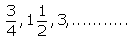Solution:

The given sequence can be rewritten as,

3/4, 3/2, 3, …..

It’s seen that, the first term is (a) = 3/4

And, common ratio(r) = (3/2)/ (3/4) = 2

We know that, the general term is

tn = arn – 1

Thus,

t8 = (3/4)(2)8 – 1 = (3/4)(2)7 = 3 x 25 = 3 x 32 = 96

5. Find the 10th term of the G.P. :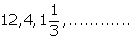Solution:

The given sequence can be rewritten as,

12, 4, 4/3, …..

It’s seen that, the first term is (a) = 12

And, common ratio(r) = (4)/ (12) = 1/3

We know that, the general term is

tn = arn – 1

Thus,

t10 = (12)(1/3)10 – 1 = (12)(1/3)9 = 12 x 1/(19683) = 4/ 6561

6. Find the nth term of the series:

1, 2, 4, 8, ……..

Solution:

It’s seen that, the first term is (a) = 1

And, common ratio(r) = 2/ 1 = 2

We know that, the general term is

tn = arn – 1

Thus,

tn = (1)(2)n – 1 = 2n – 1

Exercise 11(B) Page No: 154

1. Which term of the G.P. :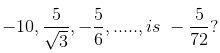Solution:

In the given G.P.

First term, a = -10

Common ratio, r = (5/√3)/ (-10) = 1/(-2√3)

We know that, the general term is

tn = arn – 1

So,

tn = (-10)( 1/(-2√3))n – 1 = -5/72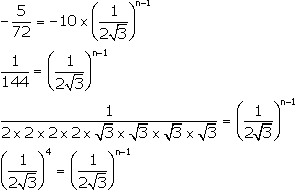Now, equating the exponents we have

n – 1 = 4

n = 5

Thus, the 5th of the given G.P. is -5/72

2. The fifth term of a G.P. is 81 and its second term is 24. Find the geometric progression.

Solution:

Given,

t5 = 81 and t2 = 24

We know that, the general term is

tn = arn – 1

So,

t5 = ar5 – 1 = ar4 = 81 …. (1)

And,

t2 = ar2 – 1 = ar1 = 24 …. (2)

Dividing (1) by (2), we have

ar4/ ar = 81/ 24

r3 = 27/ 8

r = 3/2

Using r in (2), we get

a(3/2) = 24

a = 16

Hence, the G.P. is

16, 24, 36, 54, ……

3. Fourth and seventh terms of a G.P. are 1/18 and -1/486 respectively. Find the G.P.

Solution:

Given,

t4 = 1/18 and t7 = -1/486

We know that, the general term is

tn = arn – 1

So,

t4 = ar4 – 1 = ar3 = 1/18 …. (1)

And,

t7 = ar7 – 1 = ar6 = -1/486 …. (2)

Dividing (2) by (1), we have

ar6/ ar3 = (-1/486)/ (1/18)

r3 = -1/27

r = -1/3

Using r in (1), we get

a(-1/3)3 = 1/18

a = -27/ 18 = -3/2

Hence, the G.P. is

-3/2, -3/2(-1/3), -3/2(-1/3)2, -3/2(-1/3)3, ……

-3/2, 1/2, -1/6, 1/18, …..

4. If the first and the third terms of a G.P are 2 and 8 respectively, find its second term.

Solution:

Given,

t1 = 2 and t3 = 8

We know that, the general term is

tn = arn – 1

So,

t1 = ar1 – 1 = a = 2 …. (1)

And,

t3 = ar3 – 1 = ar2 = 8 …. (2)

Dividing (2) by (1), we have

ar2/ a = 8/ 2

r2 = 4

r = ± 2

Hence, the 2nd term of the G.P. is

When a = 2 and r = 2 is 2(2) = 4

Or when a = 2 and r = -2 is 2(-2) = -4

5. The product of 3rd and 8th terms of a G.P. is 243. If its 4th term is 3, find its 7th term

Solution:

Given,

Product of 3rd and 8th terms of a G.P. is 243

The general term of a G.P. with first term a and common ratio r is given by,

tn = arn – 1

So,

t3 x t8 = ar3 – 1 x ar8 – 1 = ar2 x ar7 = a2r9 = 243

Also given,

t4 = ar4 – 1 = ar3 = 3

Now,

a2r9 = (ar3) ar6 = 243

(3) ar6 = 243

ar6 = 81

ar7 – 1 = 81 = t7

Thus, the 7th term of the G.P is 81.

Exercise 11(C) Page No: 156

1. Find the seventh term from the end of the series: √2, 2, 2√2, …… , 32

Solution:

Given series: √2, 2, 2√2, …… , 32

Here,

a = √2

r = 2/ √2 = √2

And, the last term (l) = 32

l = tn = arn – 1 = 32

(√2)( √2)n – 1 = 32

(√2)n = 32

(√2)n = (2)5 = (√2)10

Equating the exponents, we have

n = 10

So, the 7th term from the end is (10 – 7 + 1)th term from the front.

i.e. 4th term of the G.P

Hence,

t11 = (√2)(√2)4 – 1 = (√2)(√2)3 = (√2) x 2√2 = 4

2. Find the third term from the end of the G.P.

2/27, 2/9, 2/3, ……., 162

Solution:

Given series: 2/27, 2/9, 2/3, ……., 162

Here,

a = √2

r = 2/ √2 = √2

And, the last term (l) = 32

l = tn = arn – 1 = 32

(√2)( √2)n – 1 = 32

(√2)n = 32

(√2)n = (2)5 = (√2)10

Equating the exponents, we have

n = 10

So, the 7th term from the end is (10 – 7 + 1)th term from the front.

i.e. 4th term of the G.P

Hence,

t11 = (√2)(√2)4 – 1 = (√2)(√2)3 = (√2) x 2√2 = 4

3. Find the G.P. 1/27, 1/9, 1/3, ……, 81; find the product of fourth term from the beginning and the fourth term from the end.

Solution:

Given G.P. 1/27, 1/9, 1/3, ……, 81

Here, a = 1/27, common ratio (r) = (1/9)/ (1/27) = 3 and l = 81

We know that,

l = tn = arn – 1 = 81

(1/27)(3)n – 1 = 81

3n – 1 = 81 x 27 = 2187

3n – 1 = 37

n – 1 = 7

n = 8

Hence, there are 8 terms in the given G.P.

Now,

4th term from the beginning is t4 and the 4th term from the end is (8 – 4 + 1) = 5th term (t5)

Thus,

the product of t4 and t5 = ar4 – 1 x ar5 – 1 = ar3 x ar4 = a2r7 = (1/27)2(3)7 = 3

4. If for a G.P., pth, qth and rth terms are a, b and c respectively; prove that:

(q – r) log a + (r – p) log b + (p – q) log c = 0

Solution:

Let’s take the first term of the G.P. to be A and its common ratio be R.

Then,

pth term = a ⇒ ARp – 1 = a

qth term = b ⇒ ARq – 1 = b

rth term = c ⇒ ARp – 1 = c

Now,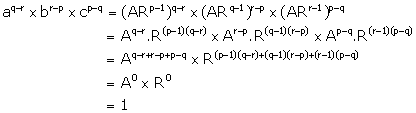On taking log on both the sides, we get

log( aq-r x br-p x cp-q ) = log 1

⇒ (q – r)log a + (r – p)log b + (p – q)log c = 0

– Hence Proved

Exercise 11(D) Page No: 156

1. Find the sum of G.P.:

(i) 1 + 3 + 9 + 27 + ………. to 12 terms

(ii) 0.3 + 0.03 + 0.003 + 0.0003 +….. to 8 terms.

(iii) 1 – 1/2 + 1/4 – 1/8 + …….. to 9 terms

(iv) 1 – 1/3 + 1/32 – 1/33 + ……… to n terms

(v)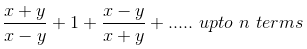(vi)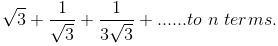Solution:

(i) Given G.P: 1 + 3 + 9 + 27 + ……….

Here,

a = 1 and r = 3/1 = 3 (r > 1)

Number of terms, n = 12

Hence,

Sn = a(rn – 1)/ r – 1

⇒ S12 = (1)((3)10 – 1)/ 3 – 1

= (312 – 1)/ 2

= (531441 – 1)/ 2

= 531440/2

= 265720

(ii) Given G.P: 0.3 + 0.03 + 0.003 + 0.0003 +…..

Here,

a = 0.3 and r = 1/10 = 0.1 (r < 1)

Number of terms, n = 8

Hence,

Sn = a(1 – rn )/ 1 – r

⇒ S8 = (0.3)(1 – 0.18 )/ (1 – 0.1)

= 0.3(1 – 0.18)/ 0.9

= (1 – 0.18)/ 3

= 1/3(1 – (1/10)8)

(iii) Given G.P: 1 – 1/2 + 1/4 – 1/8 + ……..

Here,

a = 1 and r = (-1/2)/ 1 = -1/2 (| r | < 1)

Number of terms, n = 9

Hence,

Sn = a(1 – rn )/ 1 – r

⇒ S9 = (1)(1 – (-1/2)9 )/ (1 – (-1/2))

= (1 + (1/2)9)/ (3/2)

= 2/3 x ( 1 + 1/512 )

= 2/3 x (513/512)

= 171/ 256

(iv) Given G.P: 1 – 1/3 + 1/32 – 1/33 + ………

Here,

a = 1 and r = (-1/3)/ 1 = -1/3 (| r | < 1)

Number of terms is n

Hence,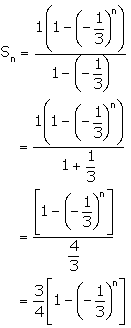Sn = a(1 – rn )/ 1 – r

(v) Given G.P:Here,

a = (x + y)/ (x – y) and r = 1/[(x + y)/ (x – y)] = (x – y)/ (x + y) (| r | < 1)

Number of terms = n

Hence,

Sn = a(1 – rn )/ 1 – r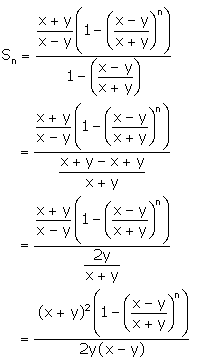(vi) Given G.P:Here,

a = √3 and r = 1/√3/ √3 = 1/3 (| r | < 1)

Number of terms = n

Hence,

Sn = a(1 – rn )/ 1 – r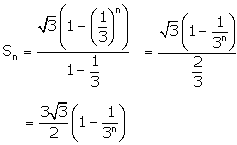2. How many terms of the geometric progression 1 + 4 + 16 + 64 + …….. must be added to get sum equal to 5461?

Solution:

Given G.P: 1 + 4 + 16 + 64 + ……..

Here,

a = 1 and r = 4/1 = 4 (r > 1)

And,

Sn = 5461

We know that,

Sn = a(rn – 1)/ r – 1

⇒ Sn = (1)((4)n – 1)/ 4 – 1

= (4n – 1)/3

5461 = (4n – 1)/3

16383 = 4n – 1

4n = 14384 = 47

n = 7

Therefore, 7 terms of the G.P must be added to get a sum of 5461.

3. The first term of a G.P. is 27 and its 8th term is 1/81. Find the sum of its first 10 terms.

Solution:

Given,

First term (a) of a G.P = 27

And, 8th term = t8 = ar8 – 1 = 1/81

(27)r7 = 1/81

r7 = 1/(81 x 27)

r7 = (1/3)7

r = 1/3 (r > 1)

Now,

Sum of first 10 terms = S10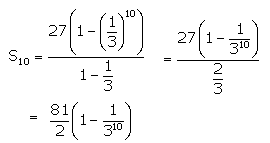4. A boy spends Rs.10 on first day, Rs.20 on second day, Rs.40 on third day and so on. Find how much, in all, will he spend in 12 days?

Solution:

Given,

Amount spent on 1st day = Rs 10

Amount spent on 2nd day = Rs 20

And amount spent on 3rd day = Rs 40

It’s seen that,

10, 20, 40, …… forms an G.P with first term, a = 10 and common ratio, r = 20/10 = 2 (r > 1)

The number of days, n = 12

Hence, the sum of money spend in 12 days is the sum of 12 terms of the G.P.

S12 = (10)(212 – 1)/ 2 – 1 = 10 (212 – 1) = 10 (4096 – 1) = 10 x 4095 = 40950

Therefore, the amount he will spend = Rs 40950

5. The 4th term and the 7th term of a G.P. are 1/27 and 1/729 respectively. Find the sum of n terms of the G.P.

Solution:

Given,

t4 = 1/27 and t7 = 1/729

We know that,

tn = arn – 1

So,

t4 = ar4 – 1 = ar3 = 1/27 …. (1)

t7 = ar7 – 1 = ar6 = 1/729 …. (2)

Dividing (2) and (1) we get,

ar6/ ar3 = (1/729)/ (1/27)

r3 = (1/3)3

r = 1/3 (r < 1)

In (1)

a x 1/27 = 1/27

a = 1

Hence,

Sn = (1 – (1/3)n )/ 1 – (1/3)

= (1 – (1/3)n )/ (2/3)

= 3/2 (1 – (1/3)n )

6. A geometric progression has common ratio = 3 and last term = 486. If the sum of its terms is 728; find its first term.

Solution:

Given,

For a G.P.,

r = 3, l = 486 and Sn = 728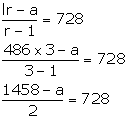1458 – a = 728 x 2 = 1456

Thus, a = 2

7. Find the sum of G.P.: 3, 6, 12, …., 1536.

Solution:

Given G.P: 3, 6, 12, …., 1536

Here,

a = 3, l = 1536 and r = 6/3 = 2

So,

The sum of terms = (lr – a)/ (r – 1)

= (1536 x 2 – 3)/ (2 – 1)

= 3072 – 3

= 3069

8. How many terms of the series 2 + 6 + 18 + ….. must be taken to make the sum equal to 728?

Solution:

Given G.P: 2 + 6 + 18 + …..

Here,

a = 2 and r = 6/2 = 3

Also given,

Sn = 728

728 = (2)(3n – 1)/ 3 – 1 = 3n – 1

729 = 3n

36 = 3n

n = 6

Therefore, 6 terms must be taken to make the sum equal to 728.

9. In a G.P., the ratio between the sum of first three terms and that of the first six terms is 125: 152.

Find its common ratio.

Solution:

Given,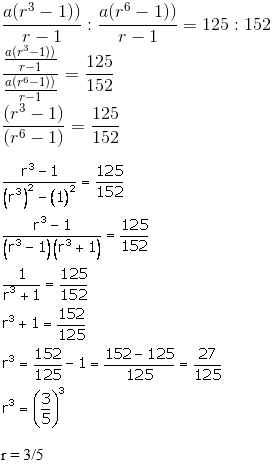Therefore, the common ratio is 3/5.

The given solutions are as per the 2019-20 Concise Selina textbook. The Selina Solutions for the academic year 2020-21 will be updated soon.